## How to get first word from text string

This post will guide you on how to extract the first word from a text string in Excel. Sometimes, you may have a long string of text and only need the first word for analysis or sorting purposes. Fortunately, there is a simple formula that can do this, or you can use VBA code to create a more powerful and flexible solution.

In this post, we will explore both methods so that you can choose the one that best suits your needs.

## 1. Get First Word using Formula in Excel

If you want to extract the first word from a text string in a cell, you can use a combination of the IF function, the ISERR function, the LEFT function and the FIND function to create a complex excel formula.

You can use the LEFT function to extract the first word, but the problem is that how to get the length of the first word in the text.  So you can use the FIND function to get the position of the first space character in the text in a cell, the number is then subtracted by 1 to get the length of the first word.

So Assuming that you want to get the first word in a text string in Cell B1, then you can write down an excel formula as follows:

``=IF(ISERR(FIND(" ",B1)),B1, LEFT(B1, FIND(" ",B1)-1))``

Let’s see how this formula works:

=FIND(” “,B1)-1

This formula returns the length of the first word in the text in Cell B1. It goes into the LEFT function as its num_chars argument.

=LEFT(B1, FIND(” “,B1)-1)

This formula extracts the first word in the text in cell B1. The LEFT function extracts the left-most characters and the number of characters that you need to extract is specified by the result returned by the FIND function.

= ISERR(FIND(” “,B1))

The ISERR function will check if the FIND function do not find any space character in the text, it means that it contains only one word or a blank Cell.

=IF(ISERR(FIND(” “,B1)),B1, LEFT(B1, FIND(” “,B1)-1))

If the ISERR function returns TRUE, then returns the value of Cell B1, otherwise, returns the first word of the text in Cell B1.

## 2. Get First Word using User Defined Function with VBA Code

You can create a User Defined Function in VBA code to extract the first word from a text string in Excel, here are the steps:

Step1: Open the Visual Basic Editor by pressing Alt + F11.

Step2: In the VBA Editor, go to Insert > Module to create a new module.

Step3: In the new module, enter the following code. Save the module and close the VBA Editor.

```Function GetFirstWord_Excelhow(str As String) As String
Dim arr() As String
arr = Split(str, " ")
GetFirstWord_Excelhow = arr(0)
End Function```

Step4: Go back to your Excel worksheet and enter a text string in a cell.

Step5: In a blank cell, enter the following formula:

``=GetFirstWord_Excelhow (B1)``

Where B1 is the cell containing the text string.

Step6: Press Enter to see the first word extracted from the text string.

The function splits the input string into an array of words using the space character as the delimiter, and returns the first word in the array using the index 0.

## 3. Video: Get First Word from Text String

This video will show you how to extract the first word from a text string in Excel using either a formula or VBA code.

## 4. Related Formulas

• Split Multiple Lines from a Cell into Rows
If you have multiple lines in a cell and each line is separated by line break character or press “alt + enter” key while you entering the text string into cells, and you need to extract the multiple lines into the separated rows or columns, you can use a combination with the TRIM function, the MID function, the SUBSTITUTE function, the REPT function, the LEN function to create a complex excel formula..…
• Extract nth word from text string
If you want to extract the nth word from a text string in a single cell, you can create an excel formula based on the TRIM function, the MID function, the SUBSTITUTE function, the REPT function and the LEN function..…
• Get last word from text string
If you want to get the last word from a text string, you can create an excel formula based on the RIGHT function, the LEN function, the FIND function and the SUBSTITUTE function..…
• count specific words in a cell or a range
If you want to count the number of a specific word in a single cell, you need to use the SUBSTITUTE function to remove all that certain word in text string, then using LEN function to calculate the length of the substring that without that specific word.…
• Extract word that starting with a specific character
Assuming that you have a text string that contains email address in Cell B1, and if you want to extract word that begins with a specific character “@” sign, you can use a combination with the TRIM function, the LEFT function, the SUBSTITUTE function, the MID function, the FIND function, the LEN function and the REPT function to create an excel formula.…

## 5. Related Functions

• Excel LEFT function
The Excel LEFT function returns a substring (a specified number of the characters) from a text string, starting from the leftmost character.The LEFT function is a build-in function in Microsoft Excel and it is categorized as a Text Function.The syntax of the LEFT function is as below:= LEFT(text,[num_chars])…t)…
• Excel IF function
The Excel IF function perform a logical test to return one value if the condition is TRUE and return another value if the condition is FALSE. The IF function is a build-in function in Microsoft Excel and it is categorized as a Logical Function.The syntax of the IF function is as below:= IF (condition, [true_value], [false_value])….
• Excel FIND function
The Excel FIND function returns the position of the first text string (sub string) within another text string.The syntax of the FIND function is as below:= FIND(find_text, within_text,[start_num])…
• Excel ISERR function
The Excel ISERR function returns TRUE if the value is any error value except #N/A.The syntax of the ISERR function is as below:= ISERR (value)…

## How to Remove Numbers from Text in Excel

This post will guide you how to remove all numbers from a text string in one cell in Excel. How do I strip all numeric characters from a given cell with a formula in Excel. How do I remove numbers from cells with text using a User Defined Function in Excel.

## Remove Numbers from Text Using Formula

Assuming that you have a list of data in range B1:B4 which contain test string values. And you want to look for a formula to remove all numbers from text string in given range of cells. You can use an array formula based on the TEXTJOIN function. Like this:

`=TEXTJOIN("",TRUE,IF(ISERR(MID(B1,ROW(INDIRECT("1:100")),1)+0),MID(B1,ROW(INDIRECT("1:100")),1),""))`

Type this formula into a blank cell and press Ctrl + Shift + Enter keys to change the formula as array formula.

## Remove Numbers from Text Using User Defined Function

You can also write a User Defined Function with VBA code to achieve the same result of removing all numbers from text string in the selected range of cells in Excel. Here are the steps:

#1 open your excel workbook and then click on “Visual Basic” command under DEVELOPER Tab, or just press “ALT+F11” shortcut.#2 then the “Visual Basic Editor” window will appear.

#3 click “Insert” ->”Module” to create a new module.#4 paste the below VBA code into the code window. Then clicking “Save” button.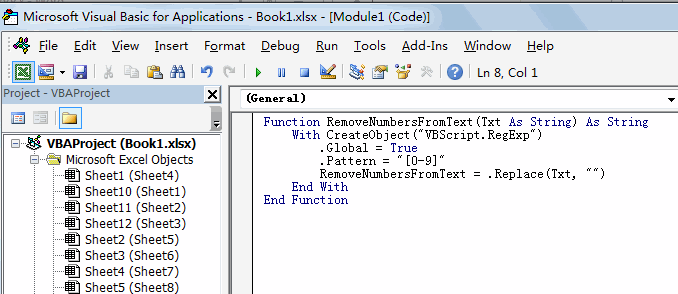#5 back to the current worksheet, then type the following formula in a blank cell, and then press Enter key.

=RemoveNumbersFromText(B1)

#6 drag the AutoFill handle over other cells to  remove numbers from text in one cell.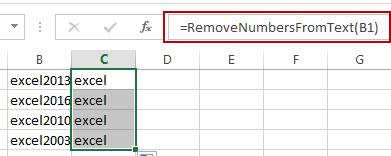### Related Functions

• Excel ROW function
The Excel ROW function returns the row number of a cell reference.The ROW function is a build-in function in Microsoft Excel and it is categorized as a Lookup and Reference Function.The syntax of the ROW function is as below:= ROW ([reference])….
• Excel IF function
The Excel IF function perform a logical test to return one value if the condition is TRUE and return another value if the condition is FALSE. The IF function is a build-in function in Microsoft Excel and it is categorized as a Logical Function.The syntax of the IF function is as below:= IF (condition, [true_value], [false_value])….
• Excel MID function
The Excel MID function returns a substring from a text string at the position that you specify.The syntax of the MID function is as below:= MID (text, start_num, num_chars)…
• Excel INDIRECT function
The Excel INDIRECT function returns the cell reference based on a text string, such as: type the text string “A2” in B1 cell, it just a text string, so you can use INDIRECT function to convert text string as cell reference….

## Removing Non Numeric Characters

This post will guide you how to remove non numeric characters from cells with a formula in Excel. How do I remove non-numeric characters in cells in Excel. How to remove non-numeric characters from cells with VBA Code in Excel. Or how to strip out non-numeric characters from a given cell in Excel.

## Removing Non Numeric Characters with a Formula

Assuming that you have a list of data in range B1:B5, and you want to remove all non-numeric characters from the range of cells B1:B5. How to achieve it. You can use an Excel Array formula based on the TEXTJOIN function, the IFERROR function, the ROW function and the INDIRECT function. Like this:

`=TEXTJOIN("",TRUE,IFERROR(MID(B1,ROW(INDIRECT("1:100")),1)+0,""))`

You need to Type this formula into a blank cell and then press CTRL +Shift + Enter keys to change the formula as an Array formula. and then drag the AutoFill Handle from C1 to C5.

## Removing Non Numeric Characters with User Defined Function

You can also try to write an user defined function with VBA code to remove all non numeric characters from a cell in Excel. Just do the following steps:

#1 open your excel workbook and then click on “Visual Basic” command under DEVELOPER Tab, or just press “ALT+F11” shortcut.#2 then the “Visual Basic Editor” window will appear.

#3 click “Insert” ->”Module” to create a new module.#4 paste the below VBA code into the code window. Then clicking “Save” button.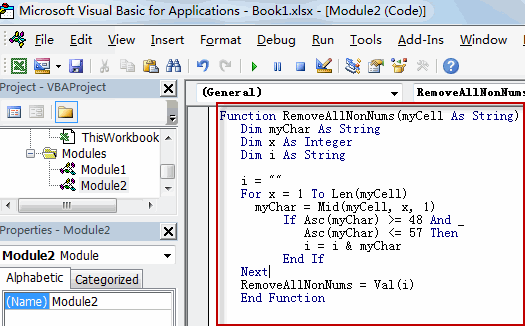```Function RemoveAllNonNums(myCell As String)
Dim myChar As String
Dim x As Integer
Dim i As String

i = ""
For x = 1 To Len(myCell)
myChar = Mid(myCell, x, 1)
If Asc(myChar) >= 48 And _
Asc(myChar) <= 57 Then
i = i & myChar
End If
Next
RemoveAllNonNums = Val(i)```

#5 back to the current worksheet, try to enter the below formula in Cell C1.

`=RemoveallNonNums(B1)`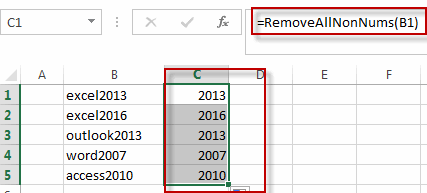You can also use the following VBA macro to achieve the same result.

```Sub RemoveAllNonNums()
Dim myR As Range
Dim myRange As Range
Set myRange = Application.Selection
Set myRange = Application.InputBox("select one range that you want to remove non-numeric characters", RemoveAllNonNums, myRange.Address, Type:=8)
For Each myR In myRange
myOut = ""
For i = 1 To Len(myR.Value)
tmp = Mid(myR.Value, i, 1)
If tmp Like "[0-9]" Then
myStr = tmp
Else
myStr = ""
End If
myOut = myOut & myStr
Next i
myR.Value = myOut
Next
End Sub```

## Removing Numeric characters only

If you only want to remove all numeric characters from a cell in Excel, and you can try to use the following array formula based on the TEXTJOIN function, the IF function, the ISERR function, the MID function, the ROW function and the INDIRECt function.

`=TEXTJOIN("",TRUE,IF(ISERR(MID(B1,ROW(INDIRECT("1:99")),1)+0),MID(B5,ROW(INDIRECT("1:99")),1),""))`

Type this formula in a blank cell and then press Ctrl + Shift + Enter shortcuts in your keyboard, and then drag the AutoFill Handle over other cells to apply this array formula.

### Related Functions

• Excel ROW function
The Excel ROW function returns the row number of a cell reference.The ROW function is a build-in function in Microsoft Excel and it is categorized as a Lookup and Reference Function.The syntax of the ROW function is as below:= ROW ([reference])….
• Excel IF function
The Excel IF function perform a logical test to return one value if the condition is TRUE and return another value if the condition is FALSE. The IF function is a build-in function in Microsoft Excel and it is categorized as a Logical Function.The syntax of the IF function is as below:= IF (condition, [true_value], [false_value])….
• Excel MID function
The Excel MID function returns a substring from a text string at the position that you specify.The syntax of the MID function is as below:= MID (text, start_num, num_chars)…
• Excel INDIRECT function
The Excel INDIRECT function returns the cell reference based on a text string, such as: type the text string “A2” in B1 cell, it just a text string, so you can use INDIRECT function to convert text string as cell reference….

## Sort Names by Middle Name in Excel

This post will guide you how to sort full name by middle name in excel 2013, or 2016. How do I sort names by middle name with excel formula. How to sort full names by middle name using Text to Columns command in excel.

## Sort Names by Middle Name using Excel Formula

Assuming that you have a list of names in your worksheet and you would like to alphabetize by middle name. You can create an excel formula based on the IF function, the ISERR function, the FIND function, and the MID function.

You need to extract the middle name firstly, then you can use the sort command to sort the middle names.  So we need to create a formula to get the middle name from the full name.

Let’s see the following example to sort full names in range B2:B4 by middle name.

1# you can write down the following formula to get middle name:

`=IF(ISERR(FIND(“ “,B2, FIND(“ “,B2)+1))),” “, MID(B2, FIND(“ “,B2)+1, FIND(“ “,B2, FIND(“ “,B2)+1))-FIND(“ “, B2)-1))`

Or you can use the following formula to get middle name:

`= MID(B2,FIND(" ",B2)+1,FIND("#",SUBSTITUTE(B2," ","#",2),1)- FIND(" ",B2,1)-1)`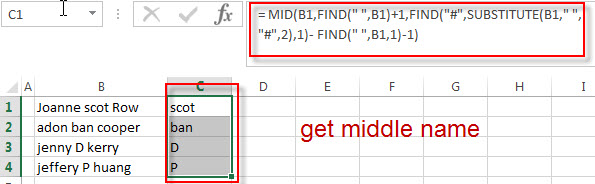If you want to know how this formula works, you can refer to this post: How to get First name, Middle name and Last name.

2# select the cells that contain middle name in range C1:D4, and then click “DATA”-> Sort A to Z.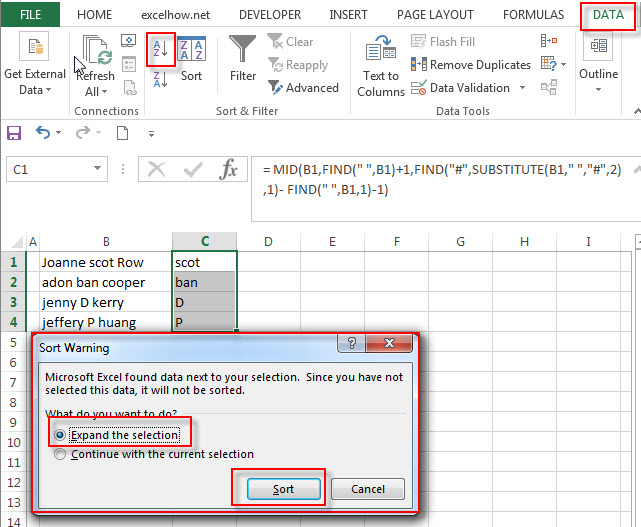3# select “Expand the selection” radio button. Then click “Sort” button. You will see that the full names have been sorted by middle names.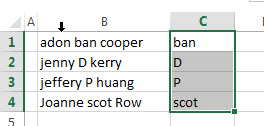## Sort Names by Middle Name using Text to Columns command

You can also use the Text to Columns command to extract the middle name from a full name in excel. Then you can sort the full name by middle name cell. Just refer to the following steps:

1# Select the range of cells that contains full name, then click on Data -> Text to Columns (under Data Tools group)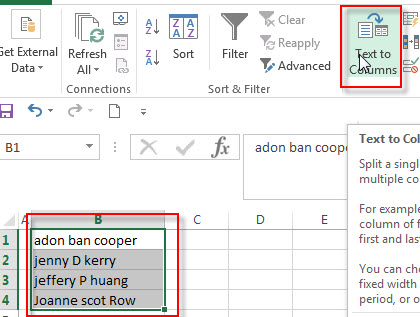2# the “Convert Text to Columns Wizard” windows will appear. Then select Delimited radio button. Click “Next” button.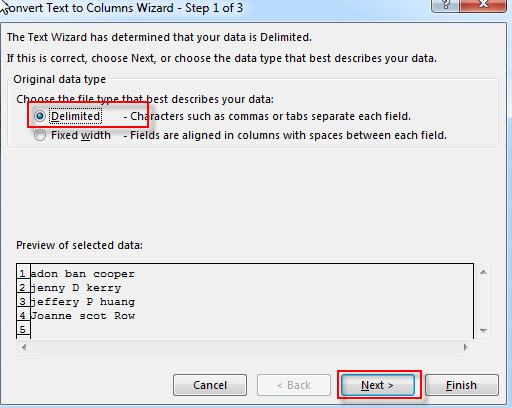3# select Space option in Delimiters section box, then click “Next” button.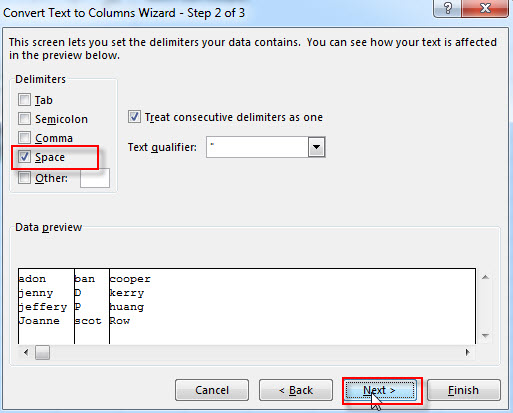4# choose Destination Cell, such as: C1, then click “Finish” button.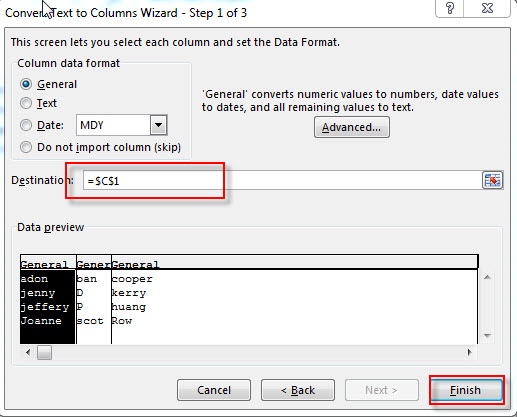5# you will see that all full names have been split into three columns.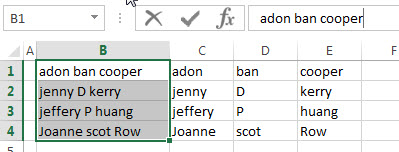6# select cells that contain middle name, then click Data-> Sort A to Z command, choose “Expand the selection” radio button, then click “sort” button.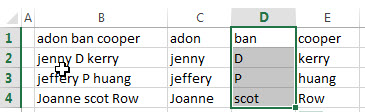### Related Posts

• Split full name to first and last name
You can create another formula based on the RIGHT function, the LEN function, the FIND function and the SUBSTITUTE function to extract the last name……
• Sort Name by Last Name in Excel
Assuming that you have a list of names in your worksheet and you would like to alphabetize by last name. You can create an excel formula based on the RIGHT function, the LEN function, the FIND function and the SUBSTITUTE function.
• Get first word from text string
If you want to extract the first word from a text string in a cell, you can use a combination of the IF function, the ISERR function, the LEFT function and the FIND function to create a complex excel formula..…
• Get last word from text string
If you want to get the last word from a text string, you can create an excel formula based on the RIGHT function, the LEN function, the FIND function and the SUBSTITUTE function..…
• Extract nth word from text string
If you want to extract the nth word from a text string in a single cell, you can create an excel formula based on the TRIM function, the MID function, the SUBSTITUTE function, the REPT function and the LEN function..…

### Related Functions

• Excel IF function
The Excel IF function perform a logical test to return one value if the condition is TRUE and return another value if the condition is FALSE. The IF function is a build-in function in Microsoft Excel and it is categorized as a Logical Function.The syntax of the IF function is as below:= IF (condition, [true_value], [false_value])….
• Excel ISERR function
The Excel ISERR function returns TRUE if the value is any error value except #N/A.The syntax of the ISERR function is as below:= ISERR (value)…
• Excel FIND function
The Excel FIND function returns the position of the first text string (sub string) within another text string.The syntax of the FIND function is as below:= FIND(find_text, within_text,[start_num])…
• Excel Substitute function
The Excel SUBSTITUTE function replaces a new text string for an old text string in a text string.The syntax of the SUBSTITUTE function is as below:= SUBSTITUTE  (text, old_text, new_text,[instance_num])….
• Excel MID function
The Excel MID function returns a substring from a text string at the position that you specify.The syntax of the MID function is as below:= MID (text, start_num, num_chars)….

## Excel ISERR Function

This post will guide you how to use Excel ISERR function with syntax and examples in Microsoft excel.

### Description

The Excel ISERR function returns TRUE if the value is any error value except #N/A.

The ISERR function is a build-in function in Microsoft Excel and it is categorized as an Information Function.

The ISERR function is available in Excel 2016, Excel 2013, Excel 2010, Excel 2007, Excel 2003, Excel XP, Excel 2000, Excel 2011 for Mac.

### Syntax

The syntax of the ISERR function is as below:

= ISERR (value)

Where the ISERR function argument is:

• Value -This is a required argument. The value that you want to test.

### Example

The below examples will show you how to use Excel ISERR Function to test if the value is any of error values(except #N/A), if so, return TRUE.

#1 = ISERR (B1)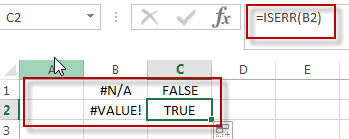### Related Functions

• Excel ISNUMBER Function
The Excel ISNUMBER function returns TRUE if the value in a cell is a numeric value, otherwise it will return FALSE.The syntax of the ISNUMBER function is as below:=ISNUMBER (value)
• Excel ISError Function
The Excel ISERROR function used to check for any error type that excel generates and it returns TRUE for any error type, such as: #N/A, #VALUE!,#REF!,#DIV0!, #NAME?, etc. The syntax of the ISERROR function is as below:= ISERROR (value)…
• Excel ISBlank Function
The Excel ISBLANK function returns TRUE if the value is blank or null.The syntax of the ISBLANK function is as below:=ISBLANK (value)…
• Excel ISNA Function
The Excel ISNA function used to check if a cell contains the #N/A error, if so, returns TRUE; otherwise, the ISNA function returns FALSE. The syntax of the ISNA function is as below:=ISNA(value)…
• Excel ISTEXT Function
The Excel ISTEXT function used to check if a value is text. If so, returns TRUE; if the text is not text, the function will return FALSE. The syntax of the ISTEXT function is as below:=ISTEXT(value)…
• Excel ISNONTEXT Function
The Excel ISNONTEXT function used to check if a value is text. If so, returns FALSE; if the text is not text, the function will return TRUE. The syntax of the ISNONTEXT function is as below:=ISNONTEXT (value)…
• Excel ISREF Function
The Excel ISREF function used to check if a value is a valid reference. If so, returns TRUE; if the value is not a reference, the function will return FALSE. The syntax of the ISREF function is as below:=ISREF(value)…

## How to Remove Numeric Characters from a Cell

This post explains that how to remove numeric characters (numbers) from a text string in one cell in excel 2016. how to remove only numeric characters but keep the non-numeric characters from a cell containing text string with an excel formula. And how to remove numbers from a cell with a user defined function in excel VBA.

## Remove numeric characters from a cell containing text

If you want to remove numeric characters from alphanumeric string, you can use the following complex array formula using a combination of the TEXTJOIN function, the MID function, the Row function, and the INDIRECT function.

`{=TEXTJOIN("",TRUE,IF(ISERR(MID(B1,ROW(INDIRECT("1":"&LEN(B1))),1)+0),MID(B1,ROW(INDIRECT("1:"&LEN(A1))),1),""))}`

Let’s see how the above array formula works:

The MID function will extract the sub strings based on the start_num and num_chars arguments. And the INDIRECT function within ROW function will returns an array list, like below:

{1,2,3…} and the length of array is the length of CELL B1.

The MID function will use the result returned by the ROW function to extract the sub string from Cell B1, as the results by the ROW function is an array, so the MID function also returns an array list. Like below format:

`{"e","x","c","e","l","2","0","1","6}`

The result returned by MID function add zero for each value in array, if the value is non-numeric value, then the ISERR function return a #VALUE error. Or return the numeric text values will be converted to number.

the IF function will check if the result returned by ISERR function, If TRUE, then return an empty string, otherwise, return that non-numeric character using the second MID function.

`{"e","x","c","e","l","","","",""}`

Last, the TEXTJOIN function will join all values and ignore the empty string.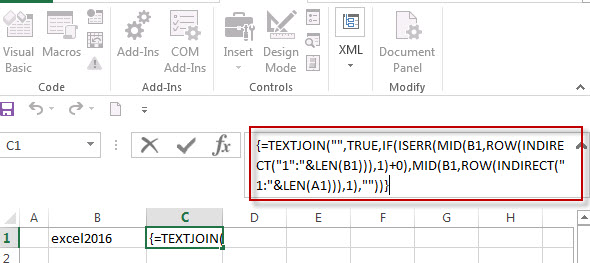## Remove numeric characters from text using a user defined function in VBA

You can create a new function to remove numeric characters from a cell that contain text string in Excel VBA. Just refer to the below steps:

1# open visual Basic Editor, then insert a module and name as : RemoveNumericCharacters.

2# click “Insert“->”Module“, then paste the following VBA code into the window: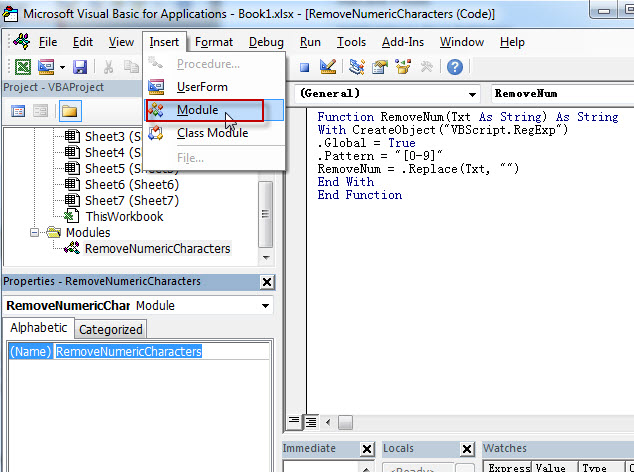```Function RemoveNum(Txt As String) As String
With CreateObject("VBScript.RegExp")
.Global = True
.Pattern = "[0-9]"
RemoveNum = .Replace(Txt, "")
End With
End Function```

3# save the user defined function.

4# enter into the below formula in Cell C1.

`=RemoveNum(B1)`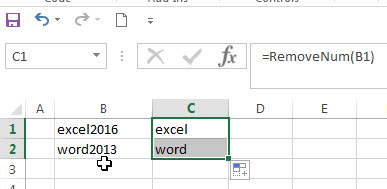### Related Formulas

• remove non numeric characters from a cell
If you want to remove non numeric characters from a text cell in excel, you can use the array formula:{=TEXTJOIN(“”,TRUE,IFERROR(MID(B1,ROW(INDIRECT(“1:”&LEN(B1))),1)+0,””))}…
• Combine Text from Two or More Cells into One Cell
If you want to combine text from multiple cells into one cell and you can use the Ampersand (&) symbol.If you are using the excel 2016, then you can use a new function TEXTJOIN function to combine text from multiple cells…

### Related Functions

• Excel TEXTJOIN function
The Excel TEXTJOIN function joins two or more text strings together and separated by a delimiter. you can select an entire range of cell references to be combined in excel 2016.The syntax of the TEXTJOIN function is as below:= TEXTJOIN  (delimiter, ignore_empty,text1,[text2])…
• Excel MID function
The Excel MID function returns a substring from a text string at the position that you specify.The syntax of the MID function is as below:= MID (text, start_num, num_chars)…
• Excel LEN function
The Excel LEN function returns the length of a text string (the number of characters in a text string). The LEN function is a build-in function in Microsoft Excel and it is categorized as a Text Function.The syntax of the LEN function is as below:= LEN(text)…
• Excel IF function
The Excel IF function perform a logical test to return one value if the condition is TRUE and return another value if the condition is FALSE.The syntax of the IF function is as below:= IF (condition, [true_value], [false_value])….
• Excel ROW function
The Excel ROW function returns the row number of a cell reference.The ROW function is a build-in function in Microsoft Excel and it is categorized as a Lookup and Reference Function.The syntax of the ROW function is as below:= ROW ([reference])….
• Excel INDIRECT  function
The Excel ROW function returns the row number of a cell reference.The ROW function is a build-in function in Microsoft Excel and it is categorized as a Lookup and Reference Function.The syntax of the ROW function is as below:= ROW ([reference])….
• Excel ISERR function
The Excel ISERR function returns TRUE if the value is any error value except #N/A.The syntax of the ISERR function is as below:= ISERR (value)…